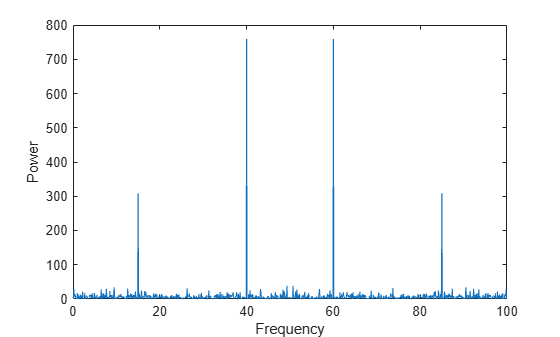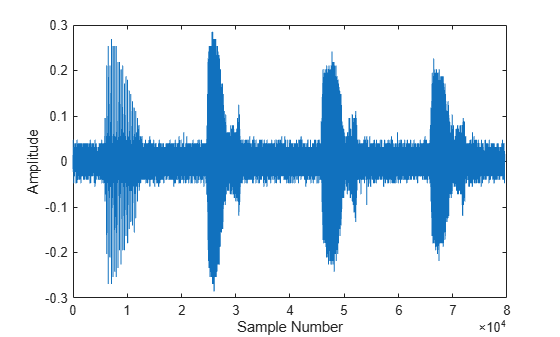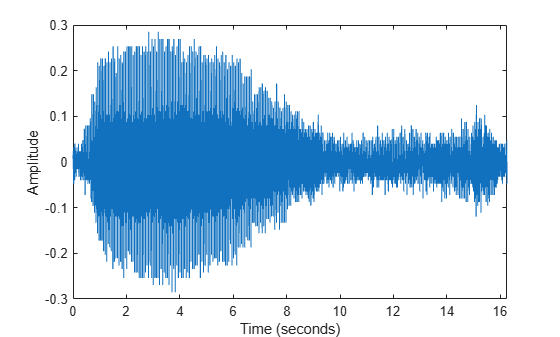Documentation

## Basic Spectral Analysis

The Fourier transform is a tool for performing frequency and power spectrum analysis of time-domain signals.

### Spectral Analysis Quantities

Spectral analysis studies the frequency spectrum contained in discrete, uniformly sampled data. The Fourier transform is a tool that reveals frequency components of a time- or space-based signal by representing it in frequency space. The following table lists common quantities used to characterize and interpret signal properties. To learn more about the Fourier transform, see Fourier Transforms.

QuantityDescription
`x`

Sampled data

`n = length(x)`

Number of samples

`fs`

Sample frequency (samples per unit time or space)

`dt = 1/fs`

Time or space increment per sample

`t = (0:n-1)/fs`

Time or space range for data

`y = fft(x)`

Discrete Fourier transform of data (DFT)

`abs(y)`

Amplitude of the DFT

`(abs(y).^2)/n`

Power of the DFT

`fs/n`

Frequency increment

`f = (0:n-1)*(fs/n)`

Frequency range

`fs/2`

Nyquist frequency (midpoint of frequency range)

### Noisy Signal

The Fourier transform can compute the frequency components of a signal that is corrupted by random noise.

Create a signal with component frequencies at 15 Hz and 40 Hz, and inject random Gaussian noise.

```fs = 100; % sample frequency (Hz) t = 0:1/fs:10-1/fs; % 10 second span time vector x = (1.3)*sin(2*pi*15*t) ... % 15 Hz component + (1.7)*sin(2*pi*40*(t-2)) ... % 40 Hz component + 2.5*gallery('normaldata',size(t),4); % Gaussian noise;```

The Fourier transform of the signal identifies its frequency components. In MATLAB®, the `fft` function computes the Fourier transform using a fast Fourier transform algorithm. Use `fft` to compute the discrete Fourier transform of the signal.

`y = fft(x);`

Plot the power spectrum as a function of frequency. While noise disguises a signal's frequency components in time-based space, the Fourier transform reveals them as spikes in power.

```n = length(x); % number of samples f = (0:n-1)*(fs/n); % frequency range power = abs(y).^2/n; % power of the DFT plot(f,power) xlabel('Frequency') ylabel('Power')```In many applications, it is more convenient to view the power spectrum centered at 0 frequency because it better represents the signal's periodicity. Use the `fftshift` function to perform a circular shift on `y`, and plot the 0-centered power.

```y0 = fftshift(y); % shift y values f0 = (-n/2:n/2-1)*(fs/n); % 0-centered frequency range power0 = abs(y0).^2/n; % 0-centered power plot(f0,power0) xlabel('Frequency') ylabel('Power')```### Audio Signal

You can use the Fourier transform to analyze the frequency spectrum of audio data.

The file `bluewhale.au` contains audio data from a Pacific blue whale vocalization recorded by underwater microphones off the coast of California. The file is from the library of animal vocalizations maintained by the Cornell University Bioacoustics Research Program.

Because blue whale calls are so low, they are barely audible to humans. The time scale in the data is compressed by a factor of 10 to raise the pitch and make the call more clearly audible. Read and plot the audio data. You can use the command `sound(x,fs)` to listen to the audio.

```whaleFile = 'bluewhale.au'; [x,fs] = audioread(whaleFile); plot(x) xlabel('Sample Number') ylabel('Amplitude')```The first sound is a "trill" followed by three "moans". This example analyzes a single moan. Specify new data that approximately consists of the first moan, and correct the time data to account for the factor-of-10 speed-up. Plot the truncated signal as a function of time.

```moan = x(2.45e4:3.10e4); t = 10*(0:1/fs:(length(moan)-1)/fs); plot(t,moan) xlabel('Time (seconds)') ylabel('Amplitude') xlim([0 t(end)])```The Fourier transform of the data identifies frequency components of the audio signal. In some applications that process large amounts of data with `fft`, it is common to resize the input so that the number of samples is a power of 2. This can make the transform computation significantly faster, particularly for sample sizes with large prime factors. Specify a new signal length `n` that is a power of 2, and use the `fft` function to compute the discrete Fourier transform of the signal. `fft` automatically pads the original data with zeros to increase the sample size.

```m = length(moan); % original sample length n = pow2(nextpow2(m)); % transform length y = fft(moan,n); % DFT of signal```

Adjust the frequency range due to the speed-up factor, and compute and plot the power spectrum of the signal. The plot indicates that the moan consists of a fundamental frequency around 17 Hz and a sequence of harmonics, where the second harmonic is emphasized.

```f = (0:n-1)*(fs/n)/10; power = abs(y).^2/n; plot(f(1:floor(n/2)),power(1:floor(n/2))) xlabel('Frequency') ylabel('Power')```